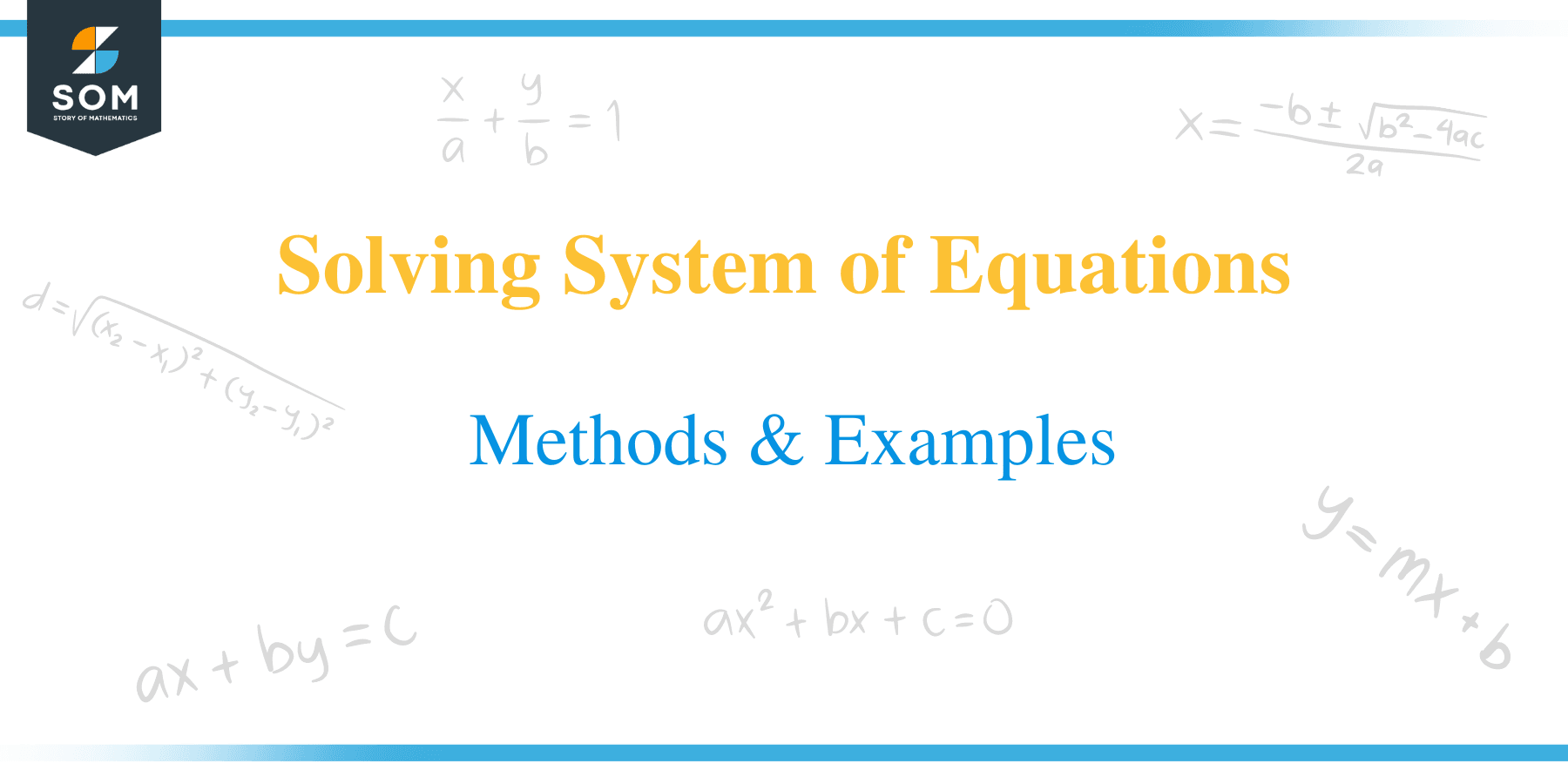# Solving System of Equations – Methods & Examples

##How to Solve System of Equations?

By now, you have got the idea of how to solve linear equations containing a single variable. What if you were when presented with multiple linear equations containing more than one variable? A set of linear equations with two or more variables is known as a system of equations.

There are several methods of solving systems of linear equations.

This article will learn how to solve linear equations using the commonly used methods, namely substitution and elimination.

### Substitution method

Substitution is a method of solving linear equations in which a variable in one equation is isolated and then used in another equation to solve for the remaining variable.

The general steps for substitution are:

• Make the subject of the formula for a variable in one of the given equations.
• Substitute the value of this variable in the second equation.’
• Solve the equation to get the value of one of the variables.
• Substitute the obtained value in any of the equations to also get the value of the other variable.Let’s solve a couple of examples using the substitution method.

Example 1

Solve the systems of equations below.

b = a + 2

a + b = 4.

Solution

Substitute the value of b into the second equation.

a + (a + 2) = 4

Now solve for a

a +a + 2 = 4

2a + 2 = 4

2a = 4 – 2

a = 2/2 = 1

Substitute the obtained value of a in the first equation.

b = a + 2

b = 1 + 2

b = 3

Hence, the solution for the two-equation is: a =1 and b=3.

Example 2

Solve the following equations using substitution.
7x – 3y = 31 ——— (i)

9x – 5y = 41 ——— (ii)

Solution

From equation (i),

7x – 3y = 31

Make y the subject of the formula in equation:

7x – 3y = 31

Subtract 7x from both sides of the equation 7x – 3y = 31 to get;

– 3y = 31 – 7x

3y = 7x – 31

3y/3 = (7x – 31)/3

Therefore, y = (7x – 31)/3

Now substitute the equation y = (7x – 31)/3 into the second equation:9x – 5y = 41

9x – 5 × (7x – 31)/3 = 41

Solving the equation gives;

27x – 35x + 155 = 41 × 3

–8x + 155 – 155 = 123 – 155

–8x = –32

8x/8 = 32/8

x = 4

By substituting the value of x in the equation y = (7x – 31)/3, we get;

y = (7 × 4 – 31)/3

y = (28 – 31)/3

y = –3/3

y = –1

Therefore, the solution to these systems of equation is x = 4 and y = –1

Example 3

Solve the following sets of equations:

2x + 3y = 9 and x – y = 3

Solution

Make x the subject of the formula in the second equation.

x = 3 + y.

Now, substitute this value of x in the first equation: 2x + 3y = 9.

⇒ 2(3 + y) + 3y = 9

⇒ 6 + 2y + 3y = 9

y = ⅗ = 0.6

Substitute the obtained value of y in the second equation – y =3.

⇒ x = 3 + 0.6

x = 3.6

Therefore, the solution is x = 3.6 and y = 0.6

### Elimination method

The following steps are followed when solving systems of equations using the elimination method:

• Equate the coefficients of the given equations by multiplying with a constant.
• Subtract the new equations common coefficients have same signs and add if the common coefficients have opposite signs,
• Solve the equation resulting from either addition or subtraction
• Substitute the obtained value in any of the equations to get the value of the other variable.Example 4

4a + 5b = 12,

3a – 5b = 9

Solution

Since the coefficients b are the same in the two equations, we vertically add the terms.

4a+3a) +(5b – 5b) = 12 + 9

7a = 21

a = 21/ 7

a = 3

substitute the obtained value of a=3 in the equation the first equation

4(3) + 5b = 12,

12 + 5b = 12

5b = 12-12

5b =0

b = 0/5 = 0

Therefore, the solution is a =3 and b = 0.

Example 5

Solve using elimination method.

2x + 3y = 9 ———–(i)

x – y = 3 ———–(ii)

Solution

Multiply the two equations by 2 and perform subtraction.

2x + 3y = 9

(-)

2x – 2y = 6

-5y = -3

y = ⅗ = 0.6

Now substitute the obtained value of y in the second equation: x – y = 3

x – 0.6 = 3

x = 3.6

Therefore, the solution is: x = 3.6 and y= 0.6

### Practice Questions

1. Solve the given system of equations:

2y + 3x = 38

y − 2x = 12

2. Solve x – y = 12 and 2x + y = 22.

3. Solve x/2 + 2/3 y = -1 and x – 1/3y = 3.

4. Solve 2a – 3/b = 12 and 5a – 7/b = 1.

5. Solve the system of equation x + 2y = 7 and 2x + 3y = 11.

6. Solve the system of equation 5x – 3y = 1 and 2x + y = -4.

7. Solve 2x – 3y = 1 and 3x – 4y = 1.

8. Solve the system of equations 3x – 5y = -23 and 5x + 3y = 7.